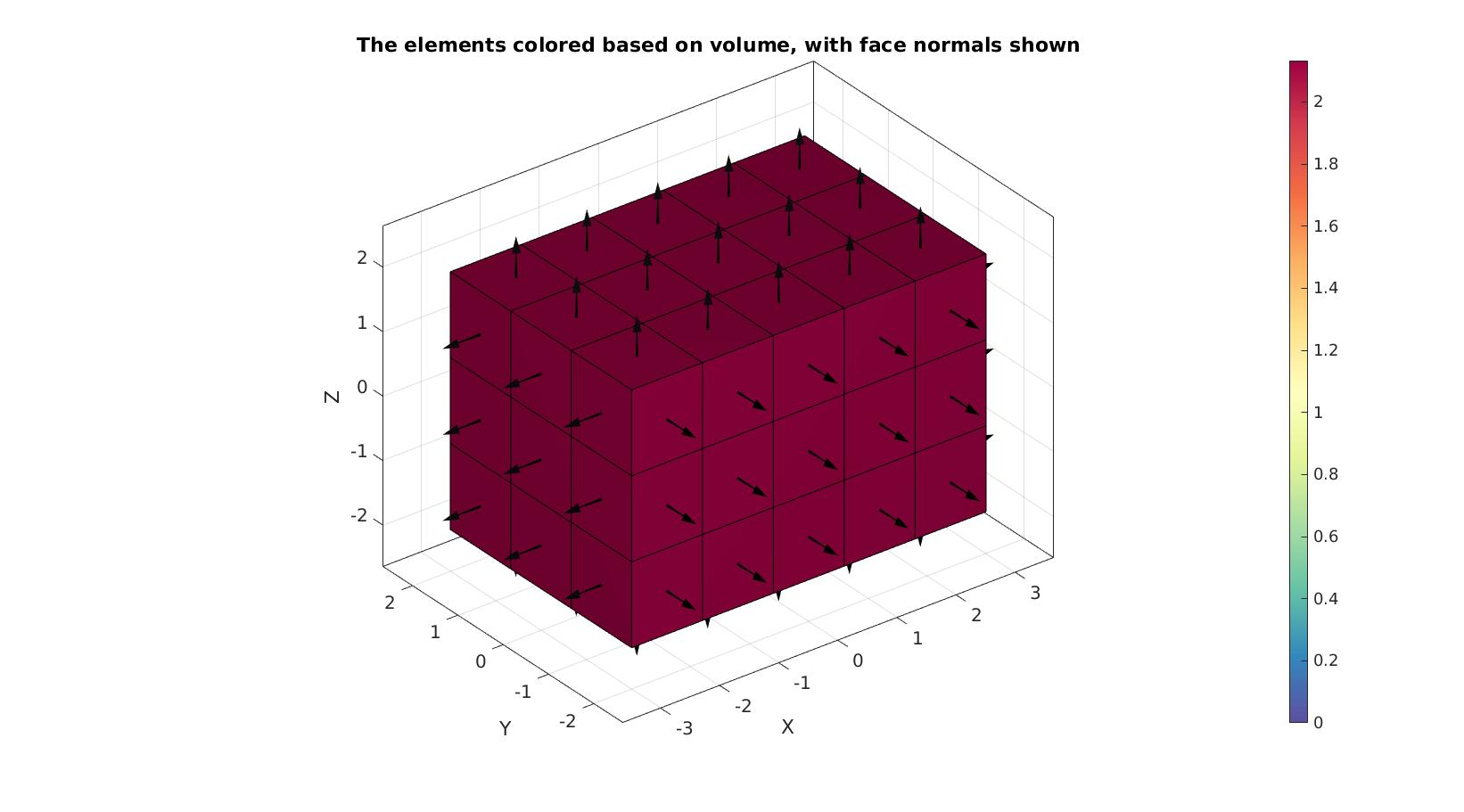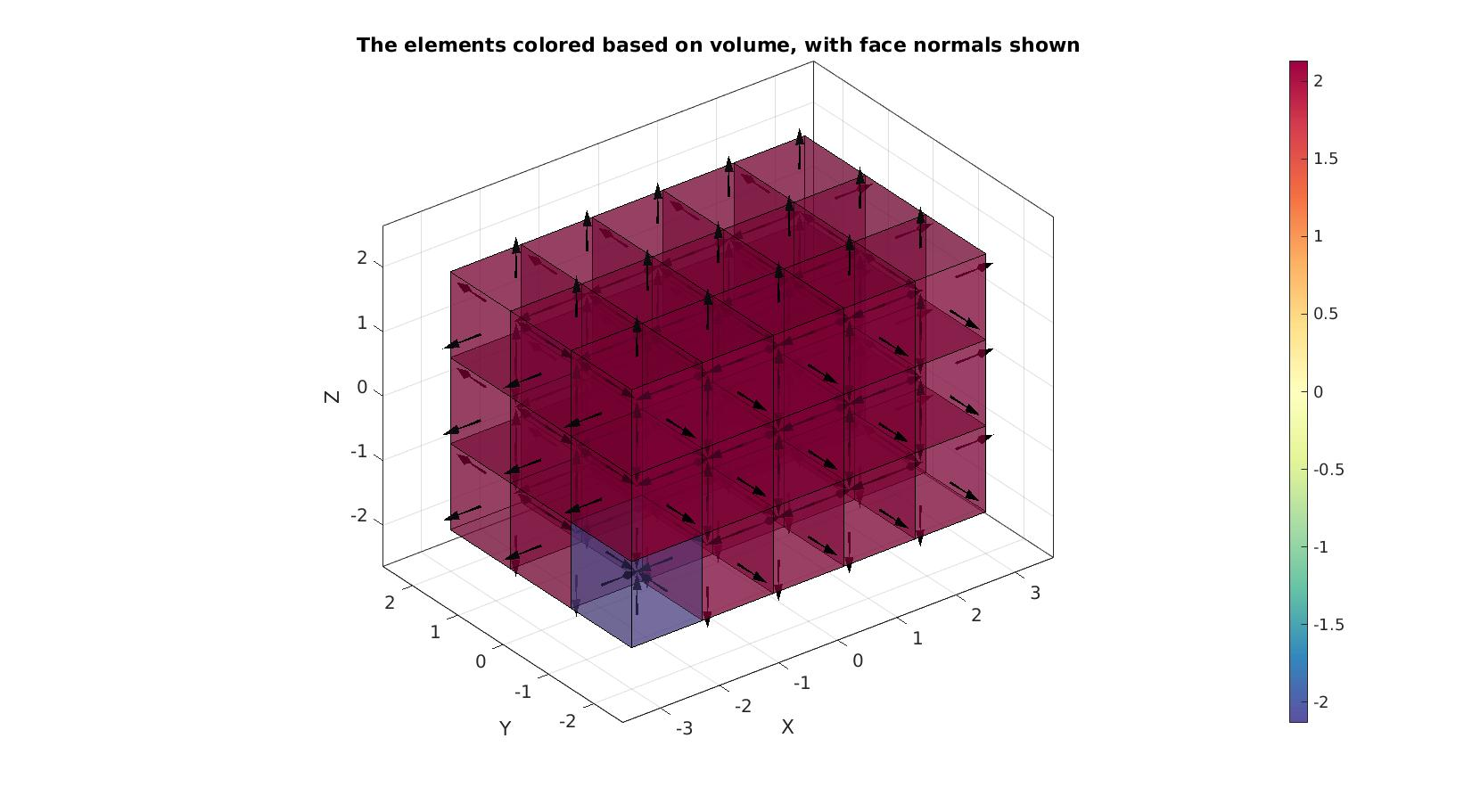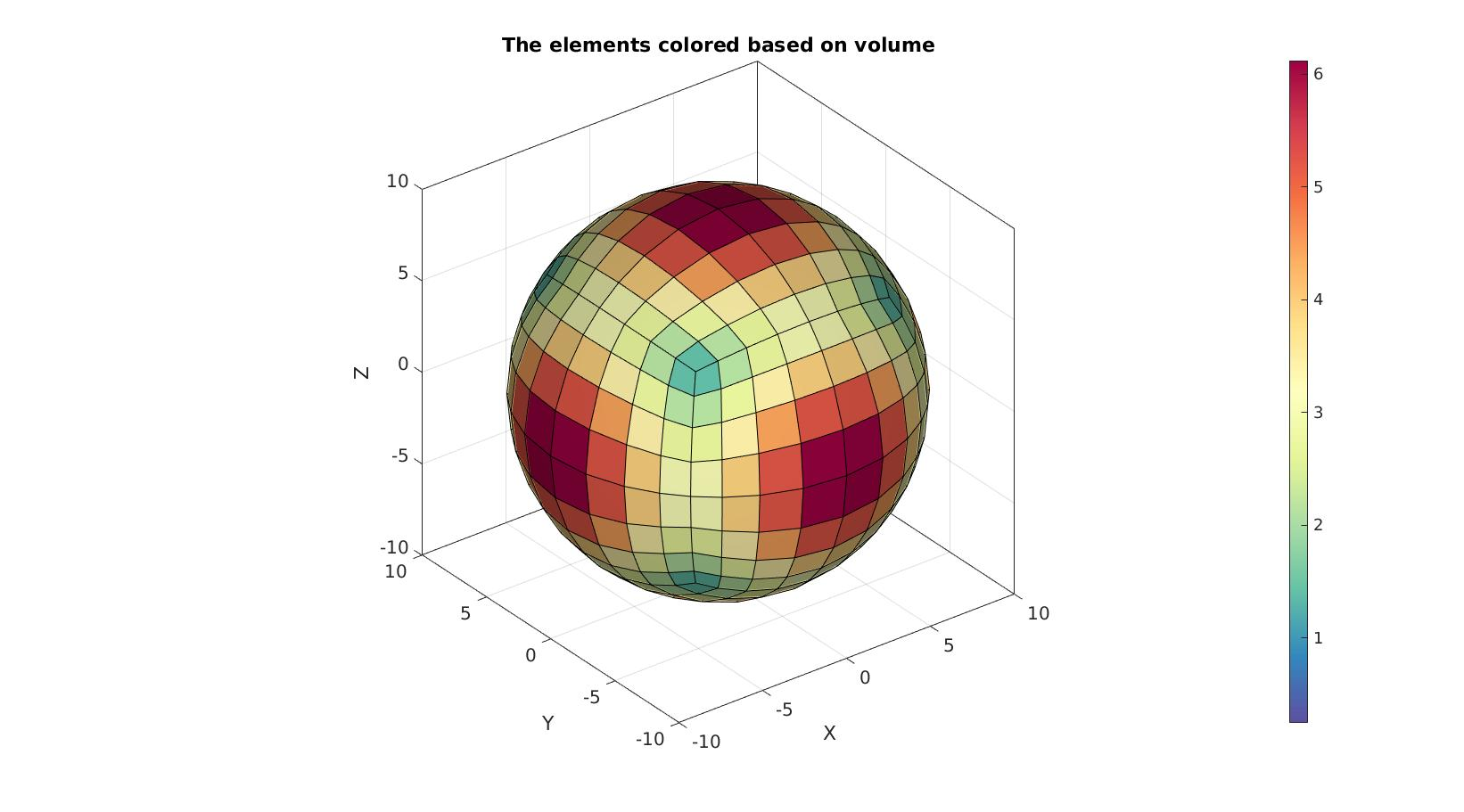# hexVol

Below is a demonstration of the features of the hexVol function

## Contents

```clear; close all; clc;
```

## Syntax

[VE,L]=hexVol(E,V,absOpt);

## Description

This function computes hexahedral element volumes. The input is the element description (E) and the nodes (V). The output is the element volumes (always positive) and a logic denoting wheter the element appears to be valid (1) or inverted (0).

## Example: Computing the volume of hexahedral elements

Create example geometry for a set of 8 hexahedral elements, each with a volume of 1, the summed volume should therefore be 8.

```%Creating a single hexahedron
X=[-1;  1; 1; -1; -1;  1; 1; -1;];
Y=[-1; -1; 1;  1; -1; -1; 1;  1;];
Z=[-1; -1;-1; -1;  1;  1; 1;  1;];
Vh=[X(:) Y(:) Z(:)];
Eh=1:8; %The hexahedral element

%Subdevided into 8 smaller elements
[E,V]=subHex(Eh,Vh,1);
```

Computing the volume

```[VE]=hexVol(E,V)
```
```VE =

1
1
1
1
1
1
1
1

```

The summed volume should be 8 for this cube

```sum(VE)
```
```ans =

8

```

Visualize mesh and face normals

```%Create patch data for plotting
[F,C]=element2patch(E,VE);

cFigure; hold on;
title('The elements colored based on volume, with face normals shown')
gpatch(F,V,C,'k',1);
patchNormPlot(F,V);
colormap spectral; colorbar;
drawnow;
```## Example: Handling negative volumes

Volumes are made absolute by default. To help detect inverted elements the optional input absOpt can be set to 0 to allow for negative volume output. In addition an output logicPositive can be requested which is true for postive volumes and false for negative volumes.

```E_inverted=E; %Copy element set
E_inverted(1,:)=E_inverted(1,[5:8 1:4]); %Invert first element

%Inspect element volumes and logic
absOpt=1 %Output absolute volumes
[VE,logicPositive]=hexVol(E_inverted,V,absOpt)

absOpt=0 %Output may include negative volumes
[VE,logicPositive]=hexVol(E_inverted,V,absOpt)
```
```absOpt =

1

VE =

1
1
1
1
1
1
1
1

logicPositive =

8×1 logical array

0
1
1
1
1
1
1
1

absOpt =

0

VE =

-1
1
1
1
1
1
1
1

logicPositive =

8×1 logical array

0
1
1
1
1
1
1
1

```

Visualize mesh and face normals

```%Create patch data for plotting
[F,C]=element2patch(E_inverted,VE);

cFigure; hold on;
title('The elements colored based on volume, with face normals shown')
gpatch(F,V,C,'k',0.5);
patchNormPlot(F,V);
colormap spectral; colorbar;
drawnow;
```## Example: A more complex hex mesh

```%Control settings
optionStruct.numElementsMantel=5;
optionStruct.numElementsCore=8;
optionStruct.makeHollow=0;
optionStruct.outputStructType=2;

%Creating sphere
[meshStruct]=hexMeshSphere(optionStruct);

% Access model element and patch data
Fb=meshStruct.facesBoundary;
Cb=meshStruct.boundaryMarker;
V=meshStruct.nodes;
E=meshStruct.elements;

absOpt=0; %Output may include negative volumes
VE=hexVol(E,V,absOpt);
```

Visualize mesh and face normals

```%Create patch data for plotting
[F,C]=element2patch(E,VE);

cFigure; hold on;
title('The elements colored based on volume')
gpatch(F,V,C,'k',1);
colormap spectral; colorbar;
drawnow;
```GIBBON www.gibboncode.org

Kevin Mattheus Moerman, [email protected]

GIBBON footer text

GIBBON: The Geometry and Image-based Bioengineering add-On. A toolbox for image segmentation, image-based modeling, meshing, and finite element analysis.

Copyright (C) 2006-2021 Kevin Mattheus Moerman and the GIBBON contributors

This program is free software: you can redistribute it and/or modify it under the terms of the GNU General Public License as published by the Free Software Foundation, either version 3 of the License, or (at your option) any later version.

This program is distributed in the hope that it will be useful, but WITHOUT ANY WARRANTY; without even the implied warranty of MERCHANTABILITY or FITNESS FOR A PARTICULAR PURPOSE. See the GNU General Public License for more details.

You should have received a copy of the GNU General Public License along with this program. If not, see http://www.gnu.org/licenses/.